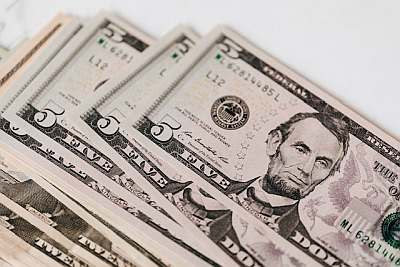# Sustainable Growth Rate Calculator

Instructions: Use this Sustainable Growth Rate Calculator to compute the sustainable growth rate $$(g)$$ by providing the retention (plow-back) ratio ($$b$$) and the return on equity $$(ROE)$$:Retention Plowback Ratio $$(b)$$ =Return on Equity $$(ROE)$$ =

## Sustainable Growth Rate Calculator

More about this sustainable growth rate calculator so you can better understand how to use this solver: The sustainable growth rate of a firm depends on the retention (plowback) ratio $$(RR)$$ and the return on equity $$(ROE)$$

### How do you calculate the sustainable growth rate?

Mathematically, the way you calculate the sustainable growth rate is by using the following formula:

$g = \displaystyle \frac{ROE \times b}{1 - ROE \times b}$

### What does sustainable growth mean?

The sustainable growth rate corresponds to the growth rate a firm can endure without increasing its level of leverage. One of the key decisions the management of a firm needs to take is the level of leverage to engage. And the sustainable growth rate shows a realistic growth rate which will no jeopardize the aimed leverage level.

### What is an appropriate growth rate?

Realistic and sustainable growth rates will strongly depend on many internal factors (capital structure, management style, etc) and external factor (industry, macroeconomic levels), so there is not one rate that is good for all firms.### Example: Calculation of the sustainable growth rate

Question: Assume that the plowback ratio for a firm is 23%, and the return on equity is 13%. Compute what would be the sustainable growth rate for this firm.

Solution:

This is the information we have been provided with:

• The retention ratio is $$b = 0.24$$ and the return on retained earnings is $$ROE = 0.13$$.

Hence, the firm's growth rate $$g$$ is computed using the following formula:

$\begin{array}{ccl} g & = & \displaystyle \frac{ROE \times b}{1 - ROE \times b} \\\\ \\\\ & = & \displaystyle \frac{0.13 \times 0.24}{1 - 0.13 \times 0.24} \\\\ \\\\ & = & 0.0322 \end{array}$

Therefore, the sustainable firm's growth is $$g = 0.0322 = 3.22\%$$, for the given retention ratio of $$b = 0.24$$ and a return on equity of $$ROE = 0.13$$.

### Other growth rates

Alternatively, you may also be interested in our growth rate calculator or our Internal Growth Rate Calculator